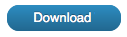# Example:- Prestressed concrete box girder bridge – Ultimate Capacity

Accompanying this are files named “ACI8280M.xsf” and “ACI8280I.xsf” that are in metric units and inch-pound units respectively.This is a cross-section of a prestressed concrete box girder bridge.

It was taken from a paper:

Troels Brondum-Nielsen, “Ultimate flexural capacity of cracked polygonal concrete sections under biaxial load“, ACI journal, title no. 82-80 November-December 1985.

The application does not uses the algorithms described in the paper although it produced results consistent with those in the paper.

An idealized rectangular stress-strain diagram was used. However it did not conform to ACI 318-89 clause 10.2.7. Thus the concrete is described as a special material.

The properties of the mild steel conform to ACI 318-89 clause 10.2.4.

The stress strain table for the prestressing steel was derived from the information in the papers; the curved parts being approximated by a sequence of straight lines. The stress values in this table were those in the paper divided by the “gamma p” factor of 1.15.

Four components were described: two shape components of Concrete called “Box” and “Deck”, a point component of Steel called “Bars”, and a point component of prestressing steel called “Tendons”.

The concrete shape was described in two parts; the bottom and the two sides being included in the component called “Box”, and the deck in the component called “Deck”.

The top right corner of the deck was adopted as the origin. This was consistent with the way the information was presented in the papers. However, as a consequence of this and the sign conventions used in the application, all of the co-ordinates were negative. (For sign conventions see the User’s Guide – “Loading” and “Distortion”.)

There were no axial loads on the cross-section. Thus the choice of the location of the reference point did not affect the meaning of the bending moment values.

The prestress “epsilon p o” value of 0.00562 given in the paper was expressed as a stage distortion in the tendons table.

Apart from this stage distortion in the tendons there were no “stage” or “other” distortions taken into account in this example. Thus in the following discussion the “Load case” distortion values quoted from the computations results would be the same as the “stress” distortion values for the concrete.

There are two Load cases in each of the accompanying xsf files.

Load case “Ultimate at 30 deg” represents the first case worked in the paper.

The angle theta described in the paper (and represented in fig. 2 in the paper) is 30 degrees. To represent this the reference angle was set to 150 degrees. That is 30 degrees from horizontal and a positive bending moment on the reference axis will have compression at the top.

The “Curvature about other axis restrained” checkbox was not checked.

The “Ultimate Bending moment” method was selected.

On the “Compute …” dialog the objective axial load is set to zero.

The computations from the “Compute ultimate bending moment” button take up to 100 or so iterations. This depends on the first estimate of the curvature deviation. The default value of zero is generally satisfactory. The default value of the curvature deviation increment of 5 degrees is also generally satisfactory. A successful computation will set the curvature deviation estimate. That can substantially reduce the number of iterations in subsequent computations.

The bending moment obtained is 5.7672 MNm. This is close to the 5.79 MNm given in the paper.

A further step shows that the distortion is consistent with the “a” and “h” given in the paper.

1. Copy the load case. This should produce a new load case called “Ultimate at 30 de 1”.
2. Change the reference angle to 180 degrees and the computation method to “Utility:- Curvature and one strain”
3. Press the compute button to obtain the “Compute” dialog.
4. Press the “Set input to current case” button. This will set the three distortion variables to represent the same distortion as obtained in the previous computation but expressed in terms of the axes at 180 degrees.

The curvature about the horizontal and vertical axes was 7.4478 x10-3 /m and -0.8170 x10-3 /m respectively.

At the origin the strain was -3.500 x10-3.

“a” is obtained from -(-3.500 x10-3)/(-0.8170 x10-3) = -4.28 m.

“h” is obtained from (-3.500 x10-3)/(7.4478 x10-3) = -0.469 m.

The Load case, “Ultimate at 0 deg.” represents the second analysis described in the paper. In this the reference angle was set to 180 degrees.

The “Curvature about other axis restrained” checkbox was checked. The “restrained” could be used because both the cross-section and the loading are to be symmetrical.

The “Ultimate Bending moment” method was selected.

On the “Compute …” dialog the objective axial load is set to zero.

In this case, with the restrained checkbox checked, the trial and adjustment has only one degree of freedom and so relatively few iterations are required, say ten.

The bending moment, 5.5218 MNm is approximately the 5.54 MNm given in the paper.

The results showed that the curvature about the horizontal and vertical axes was 12.364 x10-3 /m and zero respectively. The strain at 0, 0 was -3.500 x10-3.

The zero curvature implied “a” was infinite.

“h” is obtained from (-3.500 x10-3) / (12.364 x10-3) = -0.283 metres.